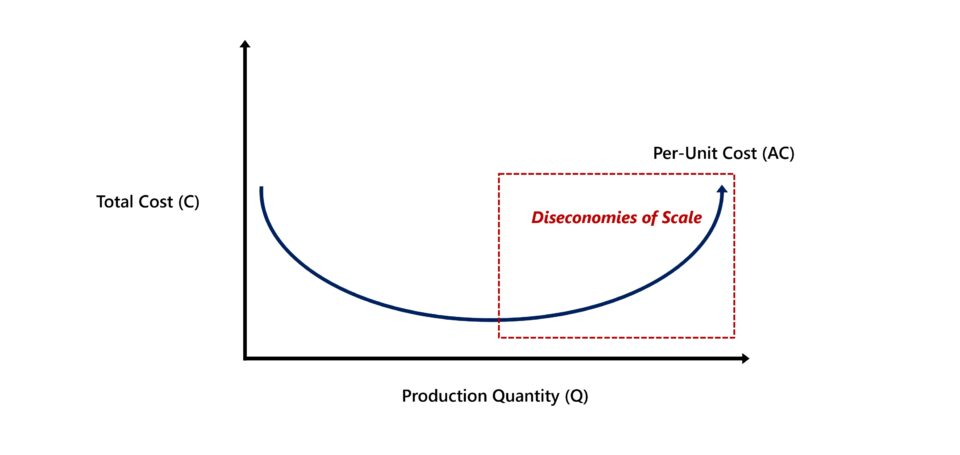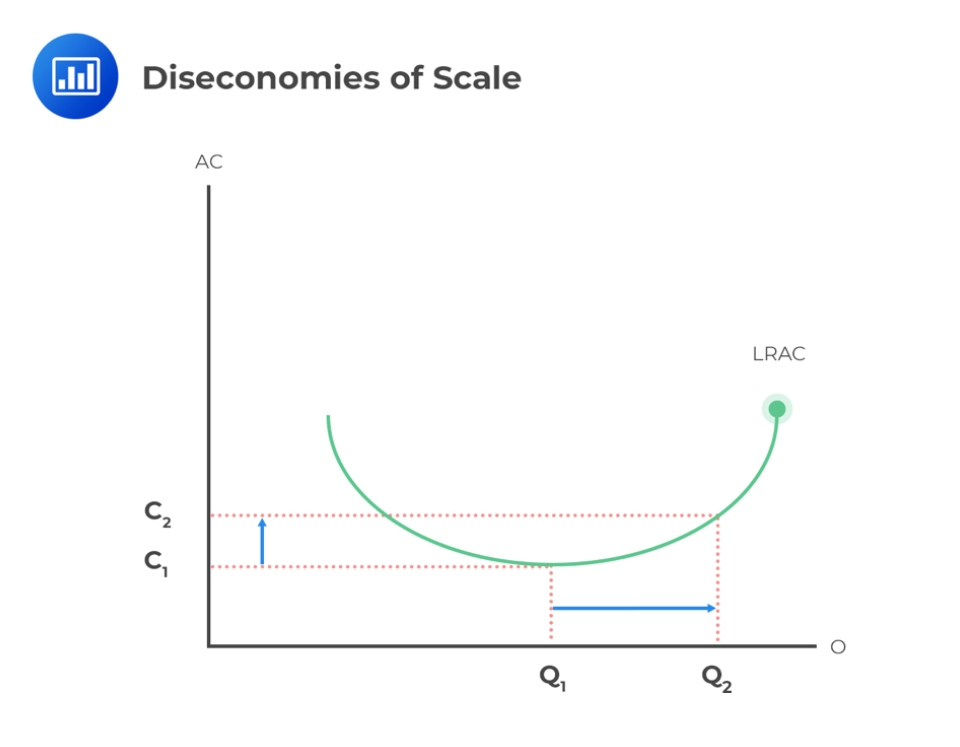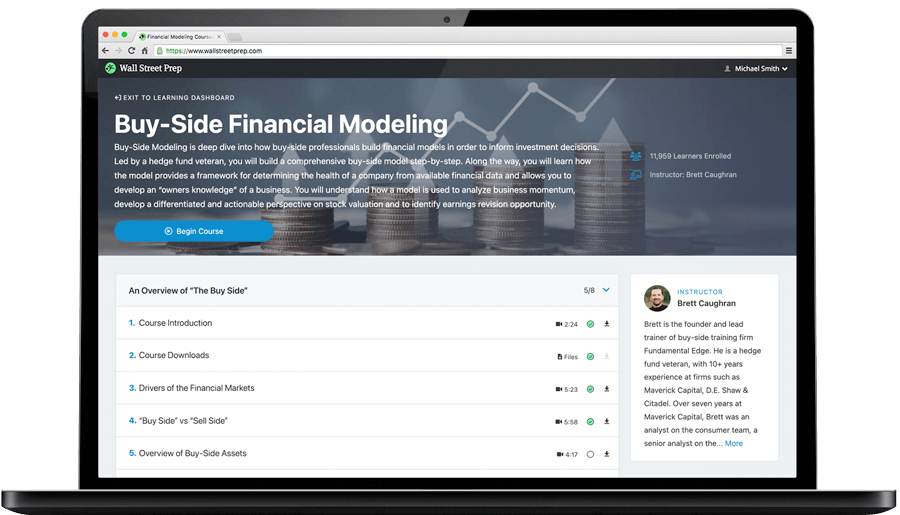Welcome to Wall Street Prep! Use code at checkout for 15% off.# Diseconomies of Scale

Guide to Understanding Diseconomies of Scale## What is the Definition of Diseconomies of Scale?

The term “diseconomies of scale” refers to a situation wherein the cost per unit of production incurred by a firm increases with a greater quantity of production output.

Generally, increased scalability and production capacity are perceived as positive factors that will contribute towards more revenue growth and profitability. However, the marginal benefit reaped from the incremental increase in production volume eventually reaches an inflection point, wherein the trajectory reverses course soon after.

Beyond the point of inflection, the profit margins of a company face downward pressure and decline, instead of incurring fewer costs and retaining more profits like earlier.

Since the unit cost per unit rises while the production volume expands, the company’s competitive positioning (and long-term profitability) is then at risk from external threats in the market, namely from the threat of new entrants.

## What Causes of Diseconomies of Scale?

The cause of diseconomies of scale can rarely be attributed to one specific factor, but the following list outlines the most common catalysts that often initiate a “domino effect” that negatively affects the financial state of a company.

• Strategic Mistakes by Management Team
• Loss of Control in Organizational Structure
• Technical Difficulties
• Misalignment in Production Capacity and Market Demand (i.e. Capacity Constraint)
• Operational Disruption (“Bottlenecks”)
• Ineffective Communication Between Divisions
• Overlap in Business Functions (or Divisions)
• Loss of Employee Morale
• Reduction in Overall Workplace Productivity

While external factors, such as the prevailing economic conditions, can contribute to the occurrence of diseconomies of scale, internal factors are more frequently the source of the problem.

For example, suppose a company’s management team decides to prioritize growth and achieving scalability to reach new markets (and customers), without much consideration for the risks posed by such corporate actions.

Occasionally, adopting that sort of mindset can work, but only if the management team truly understands the risks beforehand and takes the precautionary measures to mitigate the risk.

## What is the Difference Between Diseconomies of Scale vs. Economies of Scale?

Conceptually, the difference between economies of scale and diseconomies of scale is tied to the relationship between the cost per unit and production volume, i.e. the quantity of output.

• Economies of Scale: The term “economies of scale” describes the cost advantage — i.e. the incremental “savings” — arising from an increase in the total production output. For each additional unit produced, the per-unit fixed cost declines since such costs are not tied to the volume of output, unlike variable costs.
• Increase in Production Quantity → Lower Per Unit Cost + Higher Profit Margins
• Diseconomies of Scale: In contrast, the “diseconomies of scale” phenomenon causes the per-unit cost to increase following the production of each additional unit of quantity. Therefore, rather than the company benefiting from the expansion in scale, the reverse occurs, where the incurred costs starts rising and there is more pressure placed on the company’s profit margins.
• Increase in Production Quantity → Higher Per Unit Cost + Lower Profit Margins

In short, economies of scale is a positive attribute that can help a company establish a sustainable moat that protects its profit margins over the long-term, whereas the reverse effect occurs from diseconomies of scale.

## Diseconomies of Scale Graph Example

The law of diminishing returns is an economic principle stating that the marginal benefit earned from an increase in production volume (output) eventually declines over time.

In theory, the optimal point at which the profitability of a company is maximized is when its marginal revenue (MR) is equivalent to its marginal cost (MC), i.e. the net marginal profit is zero.

Beyond the optimal point (MR = MC), the per-unit cost that had been previously declining reverses direction and starts to increase from more production quantity. Hence, the curve on the graph starts to bend in an upward trajectory (and reflects the shape of a “U”).Graph of Diseconomies of Scale (Source: AnalystPrep)

## Diseconomies of Scale Calculation Example (Per Unit Cost)

Suppose a manufacturing company produced 1,000 widgets at a total cost of production of \$10,000 in Q1-2022.

• Production Quantity (Q) = 1,000
• Total Cost (TC) = \$10,000

The per-unit cost, also known as the “average cost per unit”, can be determined by dividing the total cost incurred (TC) by the total production units (Q).

Per-Unit Cost (C) = Total Cost (TC) ÷ Production Quantity (Q)

By inserting our assumptions into the formula, we arrive at a per-unit cost of \$10.00 for the first quarter of 2022. Therefore, the manufacturer incurs \$10.00 on average for each unit produced.

• Per-Unit Cost (C) = \$10,000 ÷ 1,000 = \$10.00

In comparison, the quarterly revenue generated by the manufacturer increased from the prior period because of the continued strength in demand from customers in the market.

In order to support the increase in market demand, the manufacturer needed to expand its production capacity, or else the demand from customers would exceed its production capacity. If that were to occur, the reputation of the manufacturer would suffer, i.e. they would be perceived by customers as being unreliable.

During the next quarter, the manufacturer produced a total of 1,200 widgets, while incurring a total cost of \$15,000.

• Production Quantity (Q) = 1,200
• Total Cost (TC) = \$15,000

Like earlier, we’ll enter our assumptions into the average cost per unit formula, which comes out to \$12.50 – reflecting a net increase of \$2.50 from the preceding quarter.

• Per-Unit Cost (C) = \$15,000 ÷ 1,200 = \$12.50

On a quarterly basis, the average cost per unit rose from \$10.00 to \$12.50, implying that the manufacturer’s profit margin at the product level declined from the operating inefficiencies stemming from the operational adjustments recently implemented to support greater production volumes.Step-by-Step Online Course

### Learn Buy-Side (Hedge Fund) Modeling

Led by a former hedge fund PM (Maverick, Citadel, DE Shaw, Schonfeld), this program begins where financial modeling training ends — with a deep-dive into how buy-side analysts build financial models to make key investment decisions.